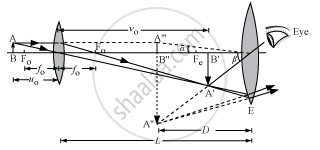Share

# A Compound Microscope Has an Objective of Focal Length 1.25 Cm and Eyepiece of Focal Length 5 Cm. a Small Object is Kept at 2.5 Cm from the Objective. - Physics

ConceptOptical Instruments The Microscope

#### Question

A compound microscope has an objective of focal length 1.25 cm and eyepiece of focal length 5 cm. A small object is kept at 2.5 cm from the objective. If the final image formed is at infinity, find the distance between the objective and the eyepiece ?

#### SolutionDistance between the objective and the eyepiece, L =

$v_0 + \left| u_e \right|$
To find v0, we have:

$u_0 = - 2 . 5 \text {cm and } f_0 = 1 . 25 cm$

$\text { Now }, - \frac{1}{u_0} + \frac{1}{v_0} = \frac{1}{f_0}$ $or \ v_0 = 2 . 5 cm$

To find ue, we have:

$v_e = \infty\text { and } f_e = 5 cm$

Calculating using the same formula as above, we get:

$u_e = - 5 cm$
∴ L = 2.5 + 5 = 7.5 cm
Is there an error in this question or solution?

#### Video TutorialsVIEW ALL 

Solution A Compound Microscope Has an Objective of Focal Length 1.25 Cm and Eyepiece of Focal Length 5 Cm. a Small Object is Kept at 2.5 Cm from the Objective. Concept: Optical Instruments - The Microscope.
S